# 1 Prostate-specific antigen (PSA)

A university medical center urology group (Stamey, et al., 1989) was interested in the association between a prostate-specific antigen (PSA) and a number of prognostic clinical measurements in men with advanced prostate cancer. Data were collected on 97 men who were about to undergo radical prostectomies. We will look at a subset of this dataset for this assignment; later in the semester we’ll be able to analyze the full complex dataset.

column Variable name Description
1 Identification number 1-97
2* PSA level Serum prostate-specific antigen level (ng/ml)
3* Cancer volume Estimate of prostate cancer volume (cc)
4* Weight Prostate weight (gm)
5* Age Age of patient (years)
6 Benign prostatic Amount of benign prostatic hyperplasia (cm2) hyperplasia
7 Seminal vesicle invasion Presence or absence of seminal vesicle invasion: 1 if yes; 0 if no
8 Capsular penetration Degree of capsular penetration (cm)
9 Gleason score Pathologically determined grade of disease (6,7,8), higher indicates worse prognosis

Background: Until recently, PSA was commonly recommended as a screening mechanism for detecting prostate cancer. To be an efficient screening tool it is important that we understand how PSA levels relate to factors that may determine prognosis and outcome. The PSA test measures the blood level of prostate-specific antigen, an enzyme produced by the prostate. PSA levels under 4 ng/mL (nanograms per milliliter) are generally considered normal, while levels over 4 ng/mL are considered abnormal (although in men over 65 levels up to 6.5 ng/mL may be acceptable, depending upon each laboratorys reference ranges). PSA levels between 4 and 10 ng/mL indicate a risk of prostate cancer higher than normal, but the risk does not seem to rise within this six-point range. When the PSA level is above 10 ng/mL, the association with cancer becomes stronger. However, PSA is not a perfect test. Some men with prostate cancer do not have an elevated PSA, and most men with an elevated PSA do not have prostate cancer. PSA levels can change for many reasons other than cancer. Two common causes of high PSA levels are enlargement of the prostate (benign prostatic hypertrophy (BPH)) and infection in the prostate (prostatitis).

# 2 Rubric

A goal here is to build a multiple regression model to predict PSA level PSA from the cancer volume V, Prostate weight Wt, and Age Age. A reasonable strategy would be to:

1. Examine the relationship between the response and the potential predictors.
2. Decide whether any of the variables should be transformed.
3. Perform a backward elimination using the desired response and predictors.
4. Given the selected model, examine the residuals and check for influential cases.
5. Repeat the process, if necessary.
6. Interpret the model and discuss any model limitations.

## 2.1(1 p) Looking at the data

Describe any patterns you see in the data. Are the ranges for each variable reasonable? Extreme/unusual observations? Strong nonlinear trends with the response suggesting a transformation?

library(tidyverse)

#   save in the same folder as this Rmd file.

str(dat_psa)
tibble [97 x 9] (S3: spec_tbl_df/tbl_df/tbl/data.frame)
$ID : num [1:97] 1 2 3 4 5 6 7 8 9 10 ...$ PSA                         : num [1:97] 0.651 0.852 0.852 0.852 1.448 ...
$cancer_volume : num [1:97] 0.56 0.372 0.601 0.301 2.117 ...$ prostate_weight             : num [1:97] 16 27.7 14.7 26.6 30.9 ...
$patient_age : num [1:97] 50 58 74 58 62 50 64 58 47 63 ...$ benign_prostatic_hyperplasia: num [1:97] 0 0 0 0 0 ...
$seminal_vesicle_invasion : num [1:97] 0 0 0 0 0 0 0 0 0 0 ...$ capsular_penetration        : num [1:97] 0 0 0 0 0 0 0 0 0 0 ...
$gleason_score : num [1:97] 6 7 7 6 6 6 6 6 7 6 ... - attr(*, "spec")= .. cols( .. ID = col_double(), .. PSA = col_double(), .. cancer_volume = col_double(), .. prostate_weight = col_double(), .. patient_age = col_double(), .. benign_prostatic_hyperplasia = col_double(), .. seminal_vesicle_invasion = col_double(), .. capsular_penetration = col_double(), .. gleason_score = col_double() .. ) #dat_psa dat_psa <- dat_psa %>% # select variables we want to use for this assignment select( ID , PSA , cancer_volume , prostate_weight , patient_age ) %>% # simplify column names rename( PSA = PSA , V = cancer_volume , Wt = prostate_weight , Age = patient_age ) str(dat_psa) tibble [97 x 5] (S3: tbl_df/tbl/data.frame)$ ID : num [1:97] 1 2 3 4 5 6 7 8 9 10 ...
$PSA: num [1:97] 0.651 0.852 0.852 0.852 1.448 ...$ V  : num [1:97] 0.56 0.372 0.601 0.301 2.117 ...
$Wt : num [1:97] 16 27.7 14.7 26.6 30.9 ...$ Age: num [1:97] 50 58 74 58 62 50 64 58 47 63 ...
summary(dat_psa)
       ID          PSA                V                 Wt              Age
Min.   : 1   Min.   :  0.651   Min.   : 0.2592   Min.   : 10.70   Min.   :41.00
1st Qu.:25   1st Qu.:  5.641   1st Qu.: 1.6653   1st Qu.: 29.37   1st Qu.:60.00
Median :49   Median : 13.330   Median : 4.2631   Median : 37.34   Median :65.00
Mean   :49   Mean   : 23.730   Mean   : 6.9987   Mean   : 45.49   Mean   :63.87
3rd Qu.:73   3rd Qu.: 21.328   3rd Qu.: 8.4149   3rd Qu.: 48.42   3rd Qu.:68.00
Max.   :97   Max.   :265.072   Max.   :45.6042   Max.   :450.34   Max.   :79.00  
library(ggplot2)
library(GGally)
#p <- ggpairs(dat_psa)
# put scatterplots on top so y axis is vertical
p <- ggpairs(dat_psa %>% select(PSA, V, Wt, Age)
#, upper = list(continuous = wrap("points", alpha = 0.2, size = 0.5))
, upper = list(continuous = "points")
, lower = list(continuous = "cor")
)
print(p)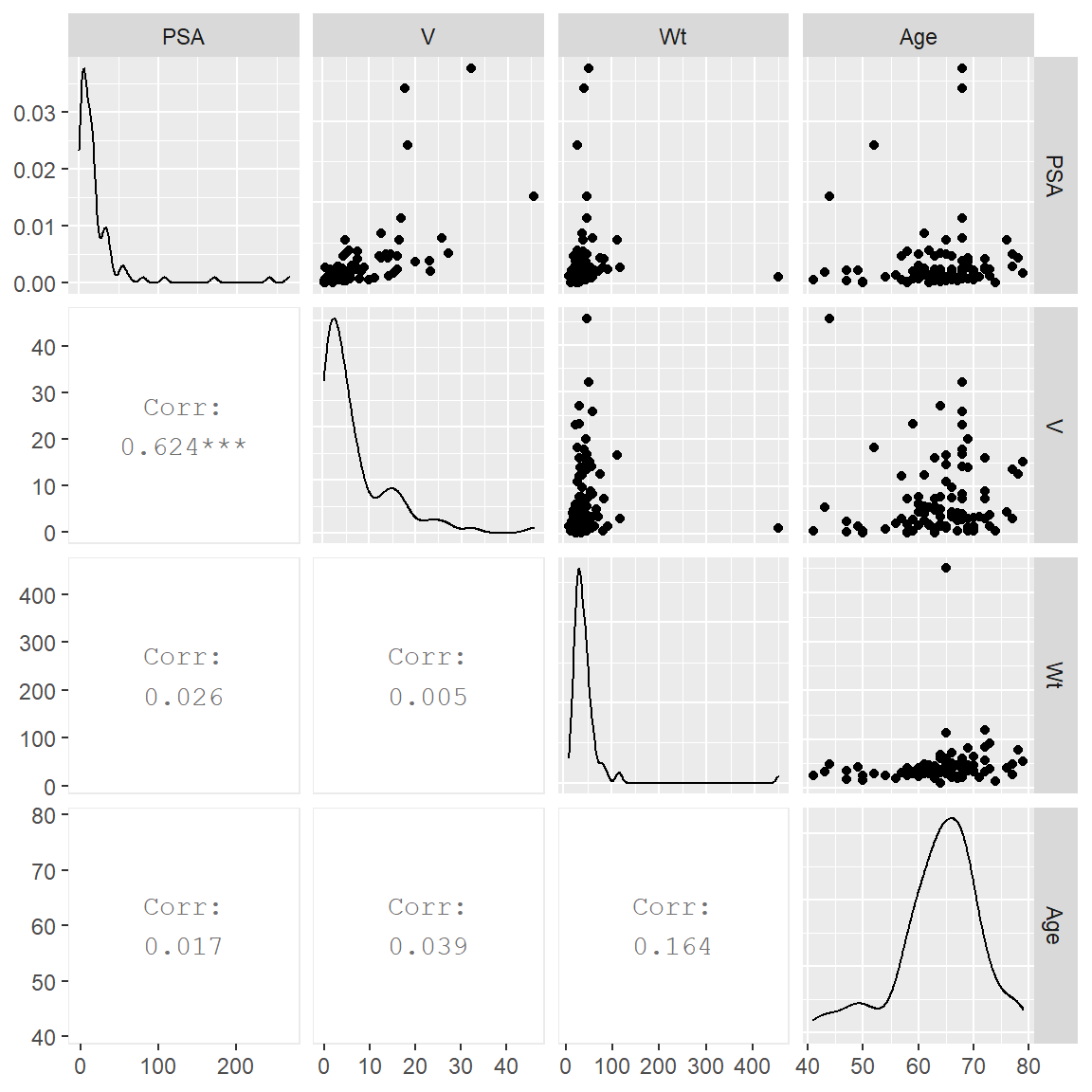# correlation matrix and associated p-values testing "H0: rho == 0"
#library(Hmisc)
dat_psa %>%
select(PSA, V, Wt, Age) %>%
as.matrix() %>%
Hmisc::rcorr()
     PSA    V   Wt  Age
PSA 1.00 0.62 0.03 0.02
V   0.62 1.00 0.01 0.04
Wt  0.03 0.01 1.00 0.16
Age 0.02 0.04 0.16 1.00

n= 97

P
PSA    V      Wt     Age
PSA        0.0000 0.7988 0.8672
V   0.0000        0.9604 0.7038
Wt  0.7988 0.9604        0.1078
Age 0.8672 0.7038 0.1078       

Bivariate scatterplots with points labeled by ID number to help characterize relationships and identify outliers.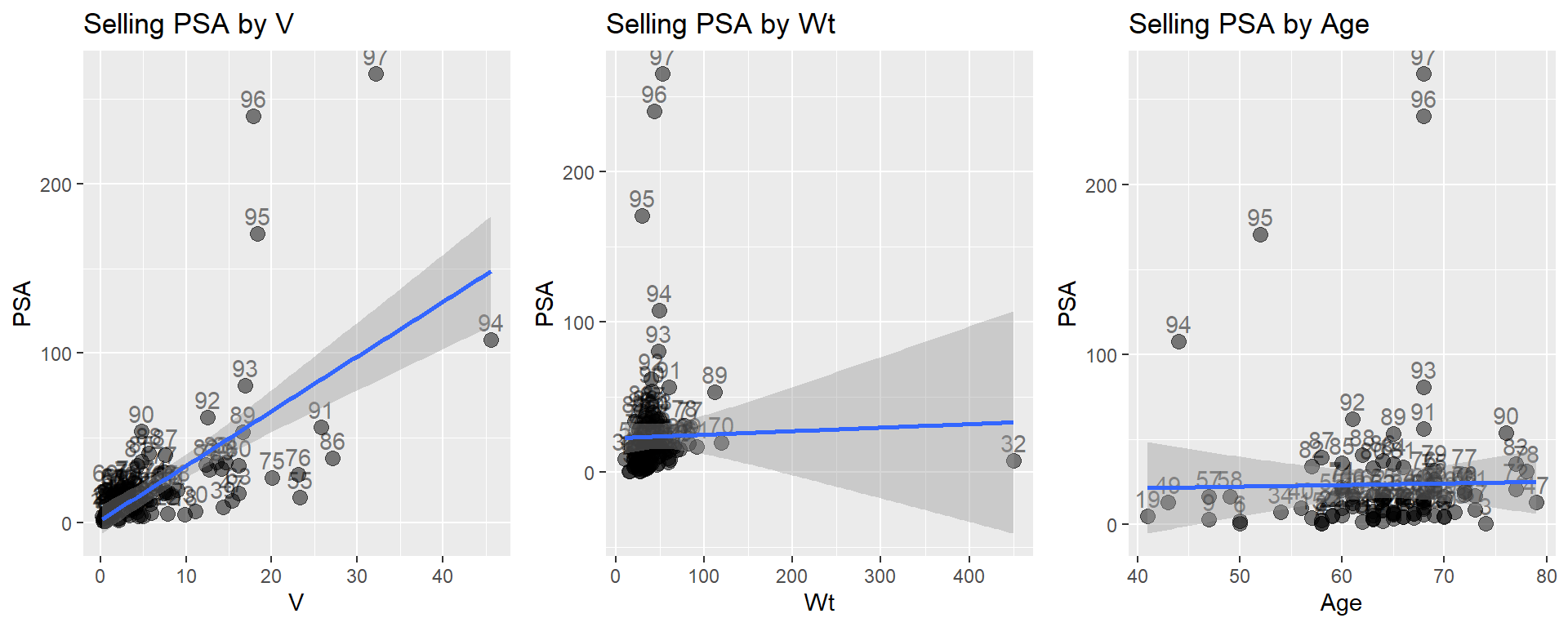## 2.2(2 p) Backward selection, diagnostics of reduced model

Fit an appropriate full model and perform backward selection using BIC. Discuss the diagnostics in terms of influential observations or problematic structure in the residuals.

### 2.2.1 Solution

Below I’ll get you started with the linear model with all the selected main effects and a set of diagnostic plots.

# fit full model
lm_psa_full <-
lm(
PSA ~ V + Wt + Age
, data = dat_psa
)

#library(car)
#Anova(aov(lm_psa_full), type=3)
summary(lm_psa_full)

Call:
lm(formula = PSA ~ V + Wt + Age, data = dat_psa)

Residuals:
Min      1Q  Median      3Q     Max
-61.687  -8.720  -1.619   3.223 181.468

Coefficients:
Estimate Std. Error t value Pr(>|t|)
(Intercept)  4.05753   28.61023   0.142    0.888
V            3.23156    0.41936   7.706 1.39e-11 ***
Wt           0.02220    0.07325   0.303    0.762
Age         -0.06191    0.45001  -0.138    0.891
---
Signif. codes:  0 '***' 0.001 '**' 0.01 '*' 0.05 '.' 0.1 ' ' 1

Residual standard error: 32.36 on 93 degrees of freedom
Multiple R-squared:  0.3902,    Adjusted R-squared:  0.3705
F-statistic: 19.84 on 3 and 93 DF,  p-value: 5.045e-10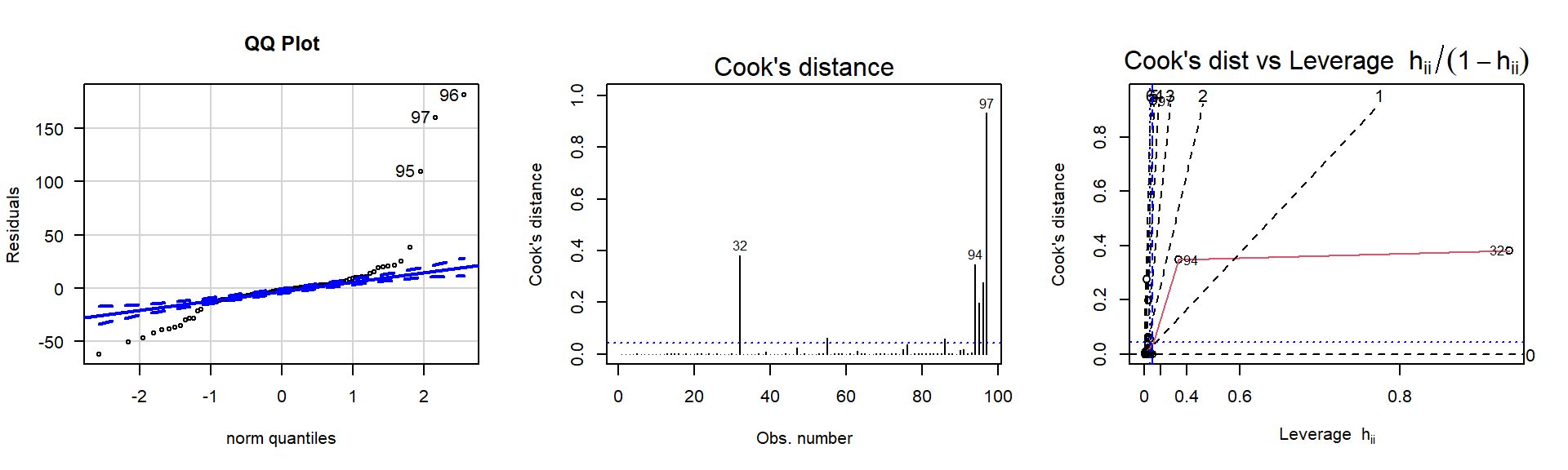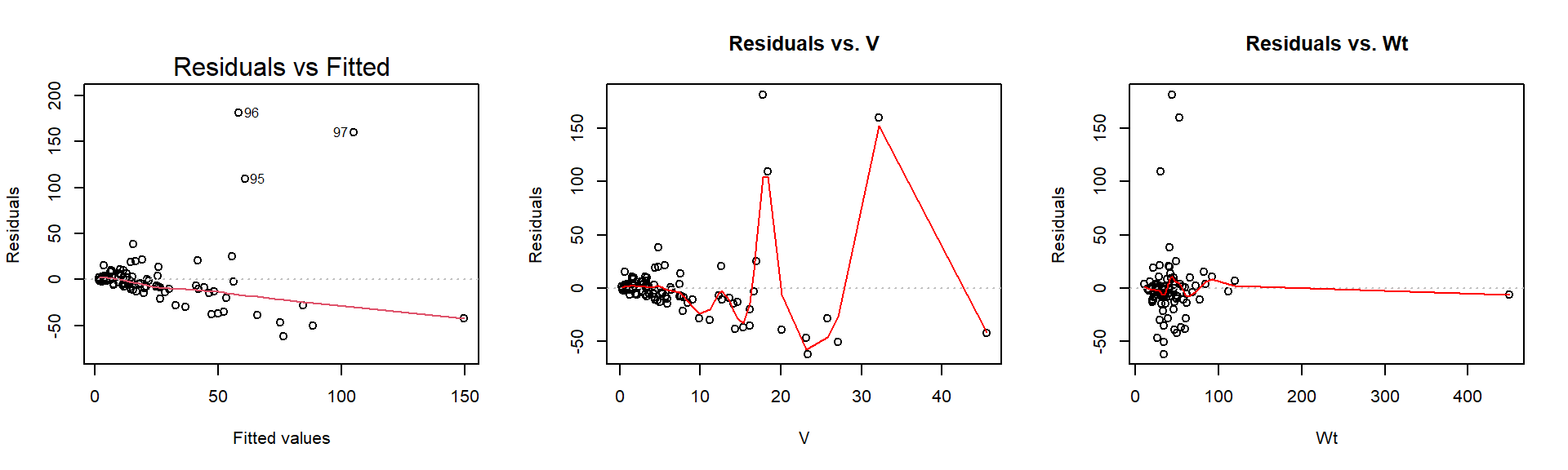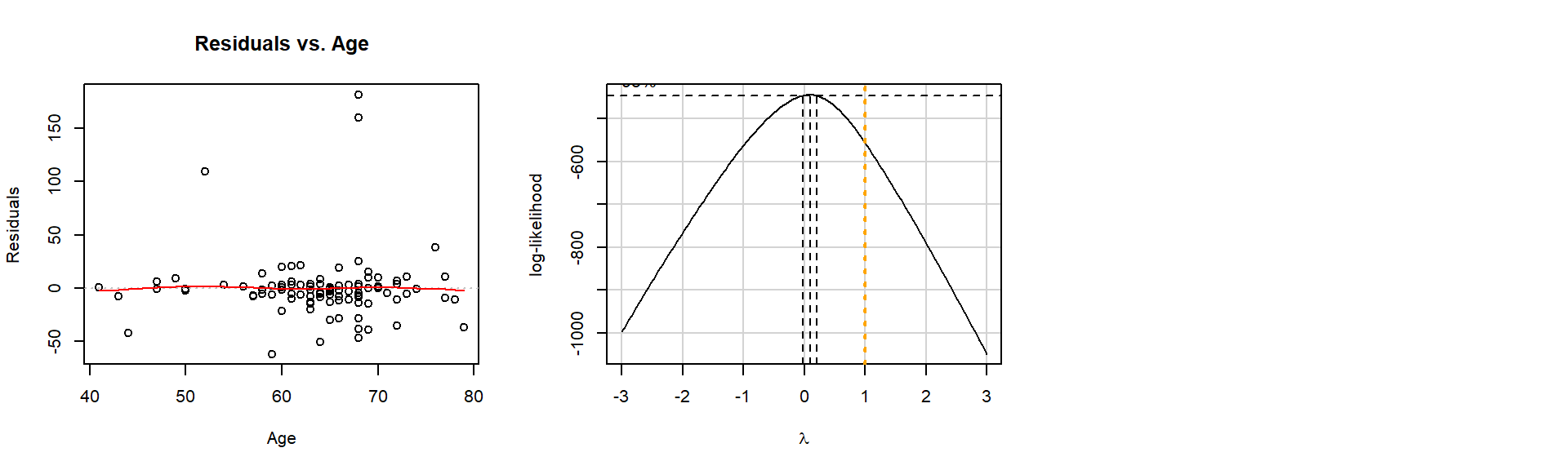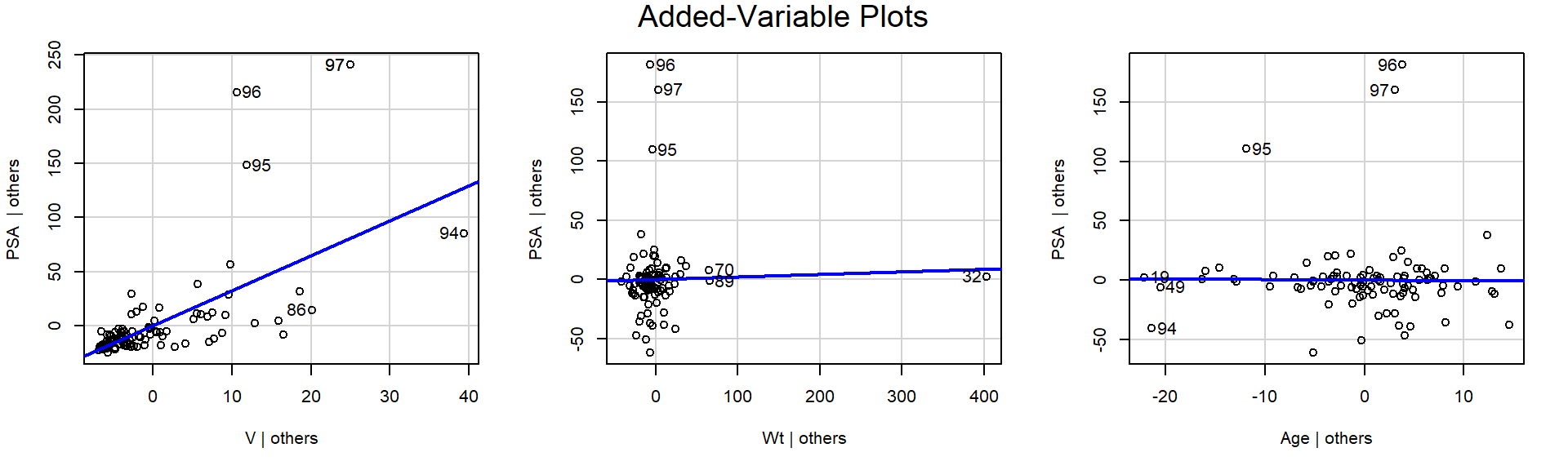Model selection starts here.

## 2.3(3 p) Address model fit

If the model doesn’t fit well (diagnostics tell you this, not $$R^2$$ or significance tests), then address the lack of model fit. Transformations and removing influential points are two strategies. The decisions you make should be based on what you observed in the residual plots. If there’s an influential observation, remove it and see how that affects the backward selection (whether the same predictors are retained), the model fit (diagnostics), and regression coefficient estimates (betas). If there’s a pattern in the residuals that can be addressed by a transformation, guess at the appropriate transformation and try it.

Repeat until you are satisfied with the diagnostics meeting the model assumptions. Below, briefly outline what you did (no need to show all the output) by (1) identifying what you observed in the diagostics and (2) the strategy you took to address that issue. Finally, show the final model and the diagnostics for that. Describe how the final model is different from the original; in particular discuss whether variables retained are different from backward selection and whether the sign and magnitude of the regression coefficients are much different.

# In the diagnostic plots, R uses the row label as the "Obs. number"
# Thus, you need to remove observations by their ID number.
#   (An ID doesn't change when a lower row is removed, but the Obs. number will.)
# Below is an example of how to do that.

# remove influential observations
dat_psa_sub <-
dat_psa %>%
filter(
!(ID %in% c( [ID numbers here] )
)

## 2.4(0 p) 3D plot

You may want to use the 3D visualization for your original final model and after making some decisions based on diagnostics. Are you convinced it’s a reasonable summary of the general relationship?

## visualize in 3D with surface
# library(rgl)
# library(car)
# scatter3d(y ~ x1 + x2, data = dat)

## 2.5(1 p) Predictive ability of the final model

What proportion of variation in the response does the model explain over the mean of the response? (This quantity indicates how precisely this model will predict new observations.)

## 2.6(2 p) Interpret the final model

Write the equation for the final model and interpret each model coefficient.